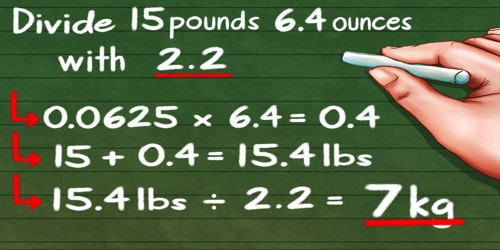How to divide pounds and ounces?

A pound is a unit of weight commonly used in the United States and the British commonwealth, and originally descended from Roman times. A pound is defined as exactly 0.45359237 kilograms.

An ounce is a unit of weight equal to 1/16th of a pound or about 28.35 grams.

How to divide pounds and ounces-

• Convert pounds and ounces to ounces by multiplying pounds by 16 and adding the number of ounces
• Perform the required division to determine the number of ounces.
• Convert the ounces to pounds and ounces by dividing by 16.
• The quotient is the number of pounds and the remainder is the number of ounces.Example: Divide 10 pounds 0 ounces by 4

Convert 10 pounds to ounces by multiplying 10 by 16:

16 * 10 = 160 ounces

Add the number of extra ounces:

160 + 0 = 160 ounces

Perform the required division:

160 ÷ 4 = 40 ounces

Convert to pounds and ounces by dividing by 16:

40 ÷ 16 = 2 R 8

The quotient (2) is the number of pounds and the remainder (8) is the number of ounces.

Basic Information:

• 16 Ounces = 1 Pound
• 14 Pounds = 1 Stone
• 2 Stones = 1 Quarter
• 4 Quarters = 1 Hundred Weight (Cwt)
• 20 Hundred Weights (Cwts) = 1 Ton

The decimal counting system uses a uniform ’10’ to advance from 1 through 10 through 100 through 1000 etc.

The process of adding and subtracting weights uses a multi-base system of 16, 14, 2, 4 and 20 to move from ounces through tons.

Information Source;